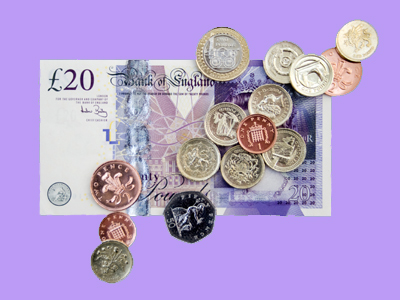Do you know all the different coins and notes we use and how much they are worth?

# Year 1 Money - Value

This quiz addresses the requirements of the National Curriculum KS1 Maths and Numeracy for children aged 5 and 6 in year 1. Specifically this quiz is aimed at the section dealing with recognising and knowing the value of money, including different denominations of coins and notes.

Children in Year 1 should begin to recognise the value of different notes and coins. This may begin by understanding what pennies are, and gradually widen to understanding the value of other coins and then notes. Eventually, children should understand that the same value can be made in several different ways, using differing combinations of coins and/or notes.

To see a larger image, click on the picture.
Question 1
What is this coin worth?
50p
£1
20p
5p
This is a £1 coin
Question 2
What is the value of the purple-coloured note?
£20
£10
20p
10p
This note has the same value as 2 x £10 notes
Question 3
What is this coin worth?
1p
10p
2p
5p
This is a 1p piece, also known as a penny
Question 4
Which coin has the lowest value in this picture?
The £1 coin
The 10p coin
The 2p coin
The 1p coin
1p has the lowest value of all the coins
Question 5
What is the highest value coin in this picture?
£1
£2
50p
20p
£2 is the highest value coin we use
Question 6
What is the value of the blue and green note in this picture?
£10
£5
£20
£50
A £5 note has the same value as 5 x £1 coins
Question 7
These are piles of...
10 pence coins
20 pence coins
5 pence coins
2 pence coins
They are 20p coins
Question 8
Which coins are not shown in this picture?
A 10p and a 1p
A 10p and a 20p
A 5p and a 2p
A 5p and a 10p
There are no £1 or £2 coins either!
Question 9
What are these coins?
5p pieces
1p pieces
10p pieces
2p pieces
These are all 2p coins
Question 10
How much money is shown here?
30p
3p
13p
33p
3 x 10p = 30p and 3 x 1p = 3p.
30p + 3p = 33p